CONTANTS

Three great constants in mathematics are pi, e, and the golden ratio (phi) which was highlighted in the book "The DaVinci Code." Below are some illustrations on how to express these constants in MAPLE to varying levels of precision.

In MAPLE, "Pi" represents the number pi and "pi" returns the Greek letter. The "evalf" command can be used to generate more or fewer digits of a floating point representation of pi.

> pi;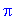> Pi;> evalf(pi);> evalf(Pi);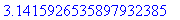> evalf(Pi, 100);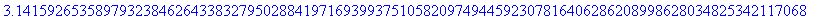To access the constant e you must evaluate the exponential function at 1 by typing "exp(1)."

> exp(1);> evalf(exp(1));> evalf(exp(1), 100);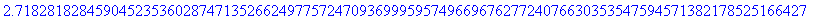You can also control the number of digits displayed by using the "Digits" command. Below, we build the number phi and set the number of digits to 100.

> phi;> phi:=(1+sqrt(5))/2;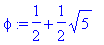> Digits:=100;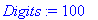> evalf(phi);>